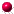###The Precedence of The Operators

If two non-assignment operators have the same precedence, they are evaluated from left to right. Thus y = 3+4+5; is evaluated as y = 7+5; or y = 12. Similarly, y = w*x/z is evaluated as y = (w*x)/z. If operators of a mixed precedence appear in an expression, the one with the highest precedence is performed from left to right, then the one with the second highest precedence is performed from left to right, and so forth. Thus z = 4/3 + 8%3 - 3; is evaluated as z = 1 + 2 -3; which is evaluated as z = 3-3; Similarly in if( a > b && c =< d), the expressions with the relational operators, a > b and c =< d, are evaluated first. Then, and only then, is the && used.

In the following table, x represents an expression. The operator with the highest precedence (the parentheses) is listed first; the one with the lowest (=), is listed last.
Operator classificationOperators
parentheses ( )
postfix operators [] x++ x--
unary operators++x --x +x -x !
creation or castnew (type)x
multiplicative* / %